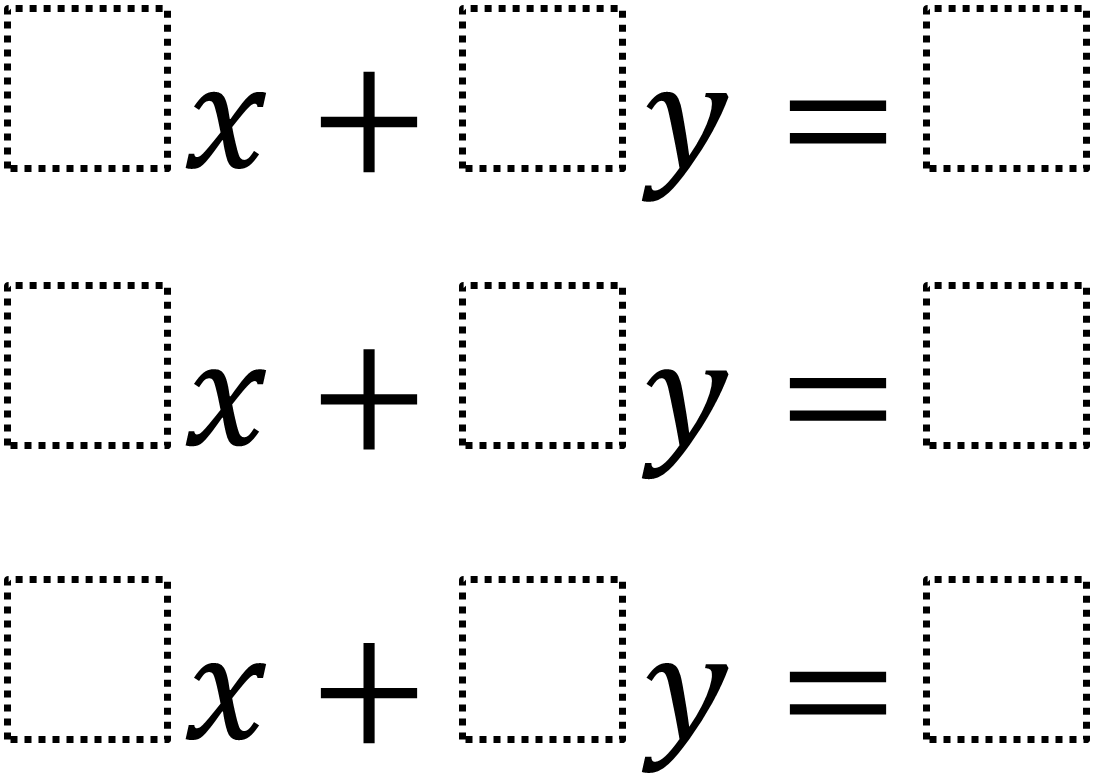# Systems of Three Equations – No Solution

Directions: Using the digits 1 through 9, at most one time each, create a system of equations that has no solutions.### Hint

What would three lines on a plane that do not intersect look like?

There are multiple answers but the lines must be parallel.  One solution is 1x + 2y = 5, 3x + 6y = 7, 4x + 8y = 9

Source: Nanette Johnson and Robert Kaplinsky

### Check Also

Directions: Directions: Using the integers from −9 to 9 at most once each, place one …

1.2.Thanks for the problem! Am I overlooking something? The way I am thinking of it, a system of 3 equations and two unknowns has a solution only if all three lines intersect at a common point. Three lines with different slopes might probably don’t do this.

1x + 2y =9
4x + 6y = 5
7x + 8y = 3

Have no common point of intersection

•No solutions would mean the three lines would have to be parallel. So they would all need the same slope.